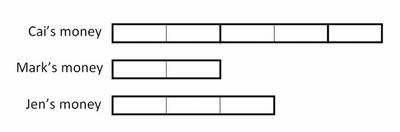# Fundraising

Alignments to Content Standards: 5.NF.B.5

Cai, Mark, and Jen were raising money for a school trip.

• Cai collected $2 \frac12$ times as much as Mark.
• Mark collected $\frac23$ as much as Jen.

Who collected the most? Who collected the least? Explain.

## Solutions

Solution: Drawing a picture

It is relatively easy to see that both Cai and Jen raised more money than Mark. The question is whether Cai made more than, the same as, or less than Jen. Drawing a picture can help.The picture shows that Cai raised $2\frac12$ as much as Mark by representing the amount that Cai made as a strip that is $2\frac12$ times as long as a strip that represents Mark's amount. Conveniently, Mark's strip is already divided into two equal pieces. Since His amount is $\frac23$ Jen's amount, we just need to add one more of those pieces to represent the total amount that Jen raised.

Cai raised $\frac52$ the amount that Mark raised.

Jen raised $\frac32$ the amount that Mark raised.

Mark raised $\frac22$ the amount that Mark raised.

Cai raised the most, Jen raised the second most, and Mark raised the least.

Solution: No picture

While more abstract, one can reason through this without a picture.

Mark raised $\frac23$ as much as Jen, so Jen raised $\frac32$ as much as Mark.

Cai raised $\frac52$ the amount that Mark raised.

Jen raised $\frac32$ the amount that Mark raised.

Mark raised $\frac22$ the amount that Mark raised.

Cai raised the most, Jen raised the second most, and Mark raised the least.